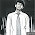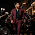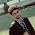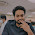TCS - C Programming Concept MCQ - Set1

1. atoi() function is used for:
a)convert ASCII character to integer value
b)convert a character string to its equivalent integer value
c)gets index value of character in an array
d)converts an array of characters to array of equivalent integers

2. Which of the following is NOT declared in string.h ?
a) strlen()
b) strcpy()
c) strptr()
d) strupr()

3. which of the below function is NOT declared in math.h ?

a) and()
b) pow()
c) exp()
d) acos()

4. Where are the local variable stored ?

a) In a Queue
b) In stack Memory
c) In hard Disk
d) In heap Memory

5. while declaring parameters for main, the second parameter argv should be declared as

a) char argv[]
b) char argv
c) char ** argv[]
d) char * argv[]

6. A memory leak happens when

a) a program allocates memory in heap but forgets to be allocate it
b) when an un-assigned pointer is used is freed using free function
c) when realloc() is called on a pointer that is not allocated
d) A program allocates memory in stack

7. what does the default header file contain?

a) Declarations
b) Implementations
c) Prototypes
d) All of the above

8. which of the below has the highest precision?

a) long int
b) double
c) unsigned long int
d) float

9. What will be the output  ( * Star Marked Question )

#include <stdio.h>
{
float f = 0.1;
if (f == 0.1)
printf("YES\n");
else
printf("NO\n");
return 0;
}
Output: NO

10. Use of ftell()

a) return current file position
b) return end of the file
c) to move file pointer position to the begining of the file
d) to flush a file

11. which of the following is used locate the end of the file ?
Mine answers was feof()

12. A code will be given and you will be asked which header is missing??

In my question sqrt() was used and math.h was missing

13. Which of the following is NOT a fundamental datatype

Ans will be enum

14. a code will be given and you have to find the error.

In my question variable r was undefined . It was star marked question so be careful

See more TCS NQT (National Qualifier Test) C-MCQ  Questions

1.ans of this qustion plz

2.3.This comment has been removed by the author.

4.ans of set 1

5.Is these are C mcq from TCS previous years or just a simple sample paper questions.

1.These are not from previous yr. , all questions were asked this year in different colleges.

6.only c language programming concepts (MCQs) are asked?

7.Till now it has been asked from C-Programming language (which also includes basics of data structure).

8.is there any chance same like questions asked in drive?

9.local variables are stored in stack

10.answer of 10th question is a. google it

11.Pls explain 10 qn